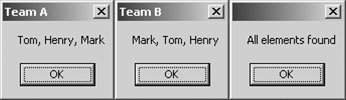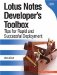# Compare Two Arrays

This function compares the values of the first array with the values of the second array. If all values in the first array are present in the second array, regardless of the order of the values, the function returns a Boolean trUE. Otherwise, the function returns a FALSE value. It's important to understand that the second array could contain additional elements that are not in the first array, and the function would still return a TRUE value as long as all of the elements in the first array are included.

How It Works

The CompareArray object will store the result of the comparison. At the start of the function, this object is set to TRUE. Next, two ForAll loops are established, one for each array. Each element in the first array is then compared to all array elements in the second array. If ElementA is found in ArrayB, then the inner loop stops and the next value in ArrayA is compared. This prevents unnecessary looping in ArrayB when a match is found. If no match is found in ArrayB, then the CompareArrays object is set to FALSE, and the function exits.

Implementation

This function requires two arrays. After the arrays are compared, the function will return either a TRUE or FALSE value.

```Function CompareArrays (ArrayA As Variant, ArrayB As Variant) As Boolean

Dim FoundMatch As Boolean
Dim ArrayMatch As Boolean
CompareArrays = True

Forall varElementA In ArrayA
FoundMatch = False
Forall varElementB In ArrayB
If varElementA = varElementB Then
FoundMatch = True
Exit Forall
End If
End Forall

If FoundMatch = False Then
CompareArrays = False
Exit Forall
End If
End Forall

End Function
```

The following code illustrates the CompareArrays function. In this example, two arrays are passed to the LotusScript library function. A message box then displays either "All elements found" (see Figure 13.4) or "All elements not found" based on the result of the array comparison.

```Sub Click(Source As Button)
Dim TeamA (0 To 2) As String
TeamA (0) = "Tom"
TeamA (1) = "Henry"
TeamA (2) = "Mark"

Dim TeamB (0 To 2) As String
TeamB (0) = "Mark"
TeamB (1) = "Tom"
TeamB (2) = "Henry"

Msgbox Implode (TeamA, ", "), , "Team A"
Msgbox Implode (TeamB, ", "), , "Team B"
If CompareArrays( TeamA, TeamB) Then
Msgbox "All elements found"
Else
End If

End Sub
```

Figure 13.4. Compare two arraysThe array values passed to the CompareArrays function can also reference fields on a form provided that the arrays are of the same type (e.g., string or numbers). In other words, an array of strings will not compare to an array of numbers. To implement this solution, simply replace the references to TeamA and TeamB with document fields as illustrated next (where FIELDA and FIELDB represent fields on a form).

```Dim w As NotesUIWorkspace
Dim s As NotesSession
Dim db As NotesDatabase
Dim uidoc As NotesUIDocument
Dim doc As NotesDocument
Set w = New NotesUIWorkspace
Set s = New NotesSession
Set db = s.CurrentDatabase
Set uidoc = w.CurrentDocument
Set doc = uidoc.Document

If CompareArrays(doc.FIELDA, doc.FIELDB) Then
Msgbox "All elements found"
Else
End If
```Lotus Notes Developers Toolbox: Tips for Rapid and Successful Deployment
ISBN: 0132214482
EAN: 2147483647
Year: N/A
Pages: 293
Authors: Mark Elliott